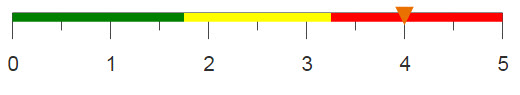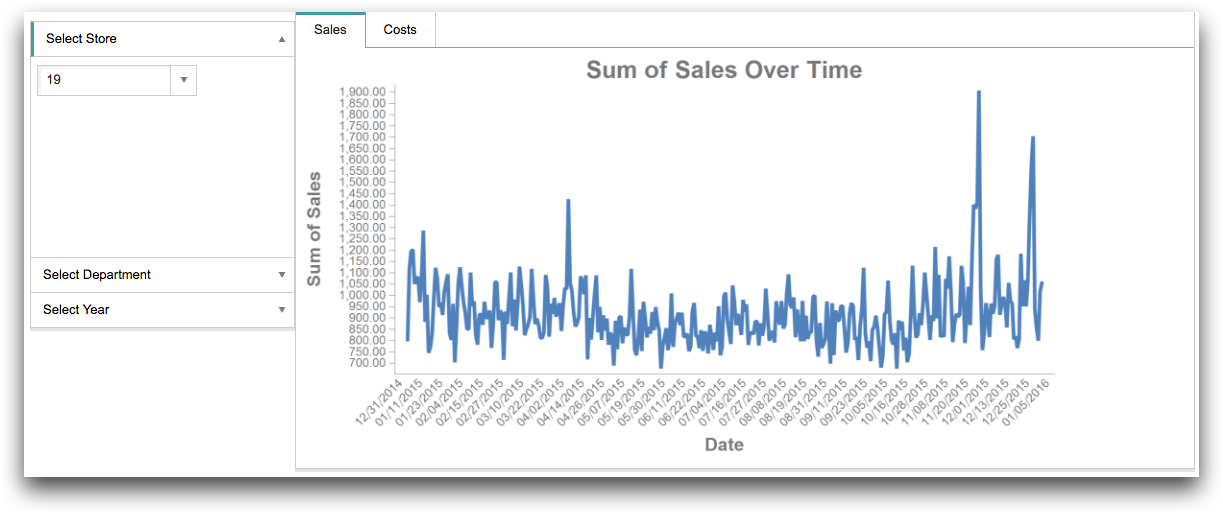# Creating layouts with accordion or collapsible panels

Using the layout type accordion (or collapsible), creates multiple panels in which additional widgets and layouts can be nested.

## Difficulty## Objective

You want to allow the user to make multiple selections but want to display each selection widget in a separate panel. By nesting each panel within a main layout of type accordion (or collapsible), each widget is displayed in a separate area, making it easier for users to keep track of which selections they've already made.

## Solution

```<dynamic store_num="19" dept_num="55" year="2015" tab_value="">
<layout arrange_="h">
<layout type_="accordion" width_="265" initopen_="0"
margin_="10px 0px 0px 10" openvalue_="@tab_value">
<layout label_="Select Store" height_="200" width_="243">
<widget name="store_num" value_="@store_num" class_="dropdown"
base_="pub.doc.retail.altseg.sales_detail_transid">
<col name="trans_date" format="type:date4y"/>
<tabu breaks="store">
<tcol source="xsales" fun="sum"/>
</tabu>
<sel value="g_first1(store;;)"/>
<colord cols="store"/>
<sort col="store" dir="up"/>
</widget>
</layout>
<layout label_="Select Department" height_="300" width_="243">
<widget name="dept_num" class_="dropdown" value_="@dept_num"
base_="pub.doc.retail.altseg.sales_detail_transid">
<col name="trans_date" format="type:date4y"/>
<tabu breaks="dept">
<tcol source="xsales" fun="sum"/>
</tabu>
<sel value="g_first1(dept;;)"/>
<colord cols="dept"/>
<sort col="dept" dir="up"/>
</widget>
</layout>
<layout label_="Select Year" height_="300" width_="243">
<widget name="year_sel" class_="checklist" inputwidth_="217"
margin_="8px 0px 0px 8" value_="@year">
<table>2014;2015
</table>
</widget>
<widget name="go_back" class_="button" type_="set" text_="Go
Back" value_="@tab_value" newvalue_="1"/>
</layout>
</layout>
<layout type_="tabpanel" width_="900">
<layout label_="Sales">
<widget name="xsales_num" class_="graphics" label_="Sales"
base_="pub.doc.retail.altseg.sales_detail_transid" width_="800">
<sel value="store={@store_num}"/>
<sel value="dept={@dept_num}"/>
<sel value="year(trans_date)={@year}"/>
<col name="trans_date" format="type:date4y"/>
<tabu breaks="trans_date">
<tcol name="xsales_sum_total" source="xsales" fun="sum"/>
</tabu>
<graphspec>
<chart type="line" title="Sum of Sales Over Time"
samples="100000">
<data x="trans_date" y="xsales_sum_total"/>
<grid hide="1"/>
<axes xlabel="Date" ylabel="Sum of Sales"/>
<ticks xrot="45" yrot="0"/>
</chart>
<style xaxissize="12" xaxislabelsize="18" yaxissize="12"/>
</graphspec>
</widget>
</layout>
<layout label_="Costs">
<widget name="cost_num" class_="graphics" label_="Cost"
base_="pub.doc.retail.altseg.sales_detail_transid" width_="800">
<sel value="store={@store_num}"/>
<sel value="dept={@dept_num}"/>
<sel value="year(trans_date)={@year}"/>
<col name="trans_date" format="type:date4y"/>
<tabu breaks="trans_date">
<tcol name="cost_sum_total" source="cost" fun="sum"/>
</tabu>
<graphspec>
<chart type="line" title="Sum of Costs Over Time"
samples="100000">
<data x="trans_date" y="cost_sum_total"/>
<grid hide="1"/>
<axes xlabel="Date" ylabel="Sum of Sales"/>
<ticks xrot="45" yrot="0"/>
</chart>
<style xaxissize="12" xaxislabelsize="18" yaxissize="12"/>
</graphspec>
</widget>
</layout>
</layout>
</layout>
</dynamic>```

## Discussion

Accordion and collapsible panels allow you to organize your widgets but still keep them all accessible to the user. These layouts are particularly useful if you want the end user to be able to make a series of selections using multiple widgets.

In this recipe, three selection widgets are created: two drop-down lists and a checklist. The selections are used to determine data displayed in two different charts. To allow the user to go through each selection more efficiently, they are all placed in different sections using type_="accordion" in the main layout. With this type, only one section can be open at a time. To allow the user to open more than one section at a time, you can replace the layout type accordion with the type collapsible.

Note that even though accordion and collapsible can be used interchangeably, there are scenarios where it is better to use one or the other. For example, if there are a plethora of different sections where opening all of them would cause the user to scroll, it is best to use accordion.

In the image below, you can see the solution using the accordion layout, and the last section is open.## Further reading

If you would like to learn more about the functions and operations discussed in this recipe, click on the links below:

<layout>